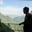Related Tags

dataframe
method
pandas
python
communitycreator

# How do we use pandas DataFrame.div() method in Python?AKASH BAJWA

## Overview

The DataFrame.div() the method in Python is used to perform division operations on the DataFrame. It is an element-wise operation and it works like a binary division ( / ) operator. It also performs floating division on the DataFrame and provides an additional feature to handle missing values.

## Syntax

DataFrame.div(other, axis = 'columns', level = None, fill_value = None)

## Parameter values

DataFrame.div() this method has four parameters in which the first parameter, other, is required while the other parameters are optional.

The parameters values for this method are as follows:

• other: This parameter is a single or multiple element data structure, or list-like object. It can be a DataFrameseries, sequence, scalar, or constant.
• axis: This is used for deciding the axis on which the operation is applied—whether to compare by the index (0 or "index") or columns (1 or "columns"), that is, {0 or ‘index’, 1 or ‘columns’}.
• level: This parameter is used for broadcasting across a level and matching index values on the passed multi-index level. A level is a number or label that indicates where to compare (point of comparison). It can either be an int or a label.
• fill_value: This argument is a number or none. It specifies what to do with NaN values before subtracting. If data in both corresponding DataFrame locations is missing, the result will also be missing.

## Return value

This method returns a dataFrame, which is obtained by the division of two DataFrames, the division of a DataFrame with a scalar, and so on.

## Example 1:

Division of each value of the DataFrame by a scalar:

# importing pandas as pd
import pandas as pd
# Creating a dataframe with five observations
df= pd.DataFrame({"ClassA":[100,50,10],
"ClassB":[50,20,30],
"ClassC":[70,70,25],
"ClassD":[150,300,0]})
# Print the dataframe
print(df)
print()
#subtractin of 10 from each and every value
print(df.div(10))
Scalar Division with Dataframe

## Explanation

• Lines 4–7: We create a DataFrame including dictionaries that have classes as keys.
• Line 9: We print the DataFrame.
• Line 12: We use the df.div(10) multiplication method with a single parameter 10 passed to it. This method divides each data value in the DataFrame.

## Example 2:

Division of the two DataFrames:

# importing pandas as pd
import pandas as pd
# Creating a dataframe with three observations
df1 = pd.DataFrame({"ClassA":[100,50,10],
"ClassB":[50,20,30],
"ClassC":[70,70,25]})

# Print the dataframe
print(df1)
print()
# Creating another dataframe
df2 = pd.DataFrame({"ClassA":[5,10,15],
"ClassB":[50,20,30],
"ClassC":[7,0,2]})

# Print the dataframe
print(df2)
print()
#
print(df2.div(df1))
Dataframes Division

## Explanation

• Lines 4–6: We create a DataFrame called df1, which includes dictionaries where the classes are the keys.
• Line 9: We print the DataFrame df1.
• Lines 12–14: We create another DataFrame called df2 on the same formatting as the other DataFrame because we are going to apply the pandas DataFrame division method to them.
• Line 17: We print the DataFrame df2.
• Line 20: We apply the method, as a result of which we obtain a DataFame that has the products of all the values of both the DataFrames, element-wise.

RELATED TAGS

dataframe
method
pandas
python
communitycreator

CONTRIBUTORAKASH BAJWA
RELATED COURSES

View all Courses

Keep Exploring

Learn in-demand tech skills in half the time